# Quasi-cyclic group

(diff) ← Older revision | Latest revision (diff) | Newer revision → (diff)

group of type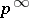An infinite Abelian-group all proper subgroups of which are cyclic (cf. Cyclic group). There exists for each prime numbera quasi-cyclic group, and it is unique up to an isomorphism. This group is isomorphic to the multiplicative group of all roots of the equations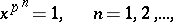in the field of complex numbers with the usual multiplication, and also to the quotient group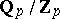, whereis the additive group of the field of rational-adic numbers andis the additive group of the ring of all-adic integers. A quasi-cyclic group is the union of an ascending chain of cyclic groupsof orders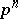,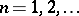; more precisely, it is the inductive limit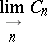with respect to the inductive system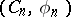. This group can be defined in terms of generators and relations as the group with countable system of generatorsand relations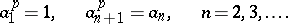Quasi-cyclic groups are the only infinite Abelian (and also the only locally-finite infinite) groups all subgroups of which are finite. The question of the existence of infinite non-Abelian groups with this property is still unsolved (1978) and constitutes one of the problems of O.Yu. Shmidt.

Quasi-cyclic groups are divisible Abelian groups (cf. Divisible group), and each divisible Abelian group is the direct sum of a set of groups that are isomorphic to the additive group of rational numbers and to quasi-cyclic groups for certain prime numbers. Groups of typeare maximal-subgroups of the multiplicative group of complex numbers, and also maximal-subgroups of the additive group of rational numbers modulo 1. The ring of endomorphisms of a group of typeis isomorphic to the ring of-adic integers. A quasi-cyclic group coincides with its Frattini subgroup.

How to Cite This Entry:
Quasi-cyclic group. Encyclopedia of Mathematics. URL: http://encyclopediaofmath.org/index.php?title=Quasi-cyclic_group&oldid=14132
This article was adapted from an original article by N.N. Vil'yams (originator), which appeared in Encyclopedia of Mathematics - ISBN 1402006098. See original article